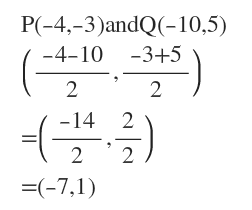# Write the equation in standard form of the conic with the given characteristics:circle: diameter with endpoints P(-4,-3) and Q(-10,5)

Question
2 views

Write the equation in standard form of the conic with the given characteristics:

circle: diameter with endpoints P(-4,-3) and Q(-10,5)

check_circle

Step 1

We find the center of the circle by finding the midpoint of P(-4,-3) and Q(-10,5)...

### Want to see the full answer?

See Solution

#### Want to see this answer and more?

Solutions are written by subject experts who are available 24/7. Questions are typically answered within 1 hour.*

See Solution
*Response times may vary by subject and question.
Tagged in